# Summary

The R package gdistance provides classes and functions to calculate various distance measures and routes in heterogeneous geographic spaces represented as grids. Least-cost distances as well as more complex distances based on (constrained) random walks can be calculated. Also the corresponding routes or probabilities of passing each cell can be determined. The package implements classes to store the data about the probability or cost of transitioning from one cell to another on a grid in a memory-efficient sparse format. These classes make it possible to manipulate the values of cell-to-cell movement directly, which offers flexibility and the possibility to use asymmetric values. The novel distances implemented in the package are used in geographical genetics (applying circuit theory), but may also have applications in other fields of geospatial analysis.

This vignette was published as an article in the Journal of Statistical Software:

van Etten, Jacob. [-@etten2017]. “R Package gdistance: Distances and Routes on Geographical Grids.” Journal of Statistical Software 76 (13): 1–21. https://doi.org/10.18637/jss.v076.i13.

# Introduction: the crow, the wolf, and the drunkard

This vignette describes gdistance, a package written for use in the R environment [@R2014]. It provides functionality to calculate various distance measures and routes in heterogeneous geographic spaces represented as grids. Distance is fundamental to geospatial analysis [@tobler1970]. It is closely related to the concept of route. For example, take the great-circle distance, the most commonly used geographic distance measure. This distance represents the shortest line between two points, taking into account the curvature of the earth. Implicit in this distance measure is a route. The great-circle distance could be conceived of as the distance measured along a route of a very efficient traveller who knows where to go and has no obstacles to deal with. In common language, this is referred to as distance as the crow flies’.

Other distance measures also imply a route across geographic space. The least-cost distance is implemented in most GIS software and mimics route finding ‘as the wolf runs’, taking into account obstacles and the local friction’ of the landscape. Since least-cost distance is affected by the environment, grid-based calculations are necessary. Other grid-based distances have been developed based on the random walk or drunkard’s walk, in which route-finding is a stochastic process.

Package gdistance was designed to determine such grid-based distances and routes and to be used in combination with other packages available within R. The gdistance package is comparable to other software such as ArcGIS Spatial Analyst [@mccoy2002], GRASS GIS [@grass], and CircuitScape [@mcrae2008]. The gdistance package also contains specific functionality for geographical genetic analyses, not found in other software yet. The package implements measures to model dispersal histories first presented by [@etten2010].

# Theory

In gdistance calculations are done in various steps. This can be confusing at first for those who are used to distance and route calculations in GIS software, which are usually done in a single step. However, an important goal of gdistance is to make the calculations of distances and routes more flexible, which also makes the package more complicated to use. Users, therefore, need to have a basic understanding of the theory behind distance and route calculations.

Calculations of distances and routes start with raster data. In geospatial analysis, rasters are rectangular, regular grids that represent continuous data over geographical space. Cells are arranged in rows and columns and each holds a value. A raster is accompanied by metadata that indicate the resolution, extent and other properties.

Distance and route calculations on rasters rely on graph theory. So as a first step, rasters are converted into graphs by connecting cell centres to each other, which become the nodes in the graph. This can be done in various ways, but only three neighbourhood graphs are commonly implemented in distance calculation software (Figure 1).

• Cells can be connected orthogonally to their four immediate neighbours, the von Neumann neighbourhood.
• Cells can be connected with their eight orthogonal and diagonal nearest neighbours, the Moore neighbourhood. The resulting graph is called the king’s graph, because it reflects all the legal movements of the king in chess. This is the most common and often only way to connect grids in GIS software.
• Connecting the cell centres in 16 directions combines king’s and knight’s moves. The function r.cost in the software GRASS [@grass] has this as an option, which inspired its implementation in gdistance. The section on distance transforms in [@deSmith2009] also discusses 16-cell neighbourhoods. Connecting in 16 directions may increase the accuracy of the calculations.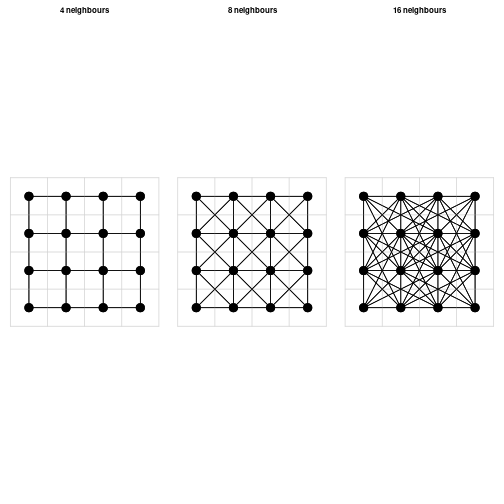When the raster is converted into a graph, weights are given to each edge (connections between nodes). These weights correspond to different concepts. In most GIS software, distance analyses are done with calculations using cost, friction or resistance values. In graph theory, weights can also correspond to conductance (1/resistance), which is equivalent to permeability (a term used in landscape ecology). The weights can also represent probabilities of transition.

Graphs are mathematically represented as matrices to do calculations. These can include transition probability matrices, adjacency matrices, resistance/conductance matrices, Laplacian matrices, among others. In gdistance, we refer collectively to these matrices to represent graphs as transition matrices’. These transition matrices are the central object in the package; all distance calculations need one or more transition matrices as an input.

In gdistance, conductance rather than resistance values are expected in the transition matrix. An important advantage of using conductance is that it makes it possible to store values in computer memory very efficiently. Conductance matrices usually contain mainly zeros, because cells are connected only with adjacent cells, and the conductance for direct connections between remote cells is zero. This makes conductance matrices suitable to store in the memory-efficient sparse matrix format. Sparse matrices do not store zero values explicitly in computer memory; they just store the non-zero values and their respective row and column indices and assume that the other values are zero. Sparse matrices do not work for resistance matrices, however, as resistance is infinite ($\infty$) between unconnected cells.

The calculation of the edge weights or conductance values is usually based on the values of the pair of grid cells to be connected. These cell values represent a property of the landscape. For instance, from a grid with altitude values, a value for the ease of walking can be calculated for each transition between cells. In gdistance, users define a function $$f(i,j)$$ to calculate the transition value for each pair of adjacent cells i and j.

With this approach, it is possible to create asymmetric matrices, in which the conductance from cell i to adjacent cell j is not always the same as the conductance from j back to i. This is relevant, among other things, for modelling travel in hilly terrain, as shown in Example 1 below. On the same slope, a downslope traveler experiences less friction than an upslope traveler. In this case, the function to calculate conductance values is non-commutative: $$f(i,j) \neq f(j,i)$$.

A problem that arises in grid-based modelling is the choice of weights that should be given to diagonal edges in proportion to orthogonal ones. For least-cost path distance and routes, this is fairly straightforward: weights are given in proportion to the distances between the cell centres. In a grid in which the orthogonal edges have a length of 1, the diagonal edges are $$\sqrt[]{2}$$ long. [@mcrae2006] applies this same idea to random walks. However, as [@birch2006] explains, for random walks this is generally not the best discrete approximation of the dispersal process in continuous space. Different orthogonal and diagonal weights could be considered based on his analytical results.

For random walks on longitude-latitude grids, there is an additional consideration to be made. Considering the eight neighbouring cells in a Moore’s neighbourhood, the three cells that are located nearer to the equator are larger in area than the three cells that are closer to the nearest pole, as the meridians converge when moving from the equator to either pole. So the cells closer to the poles should have a slightly lower probability of being reached during a random walk from the central cell. More theoretical work is needed to investigate possible solutions to this problem. For projected grids and small areas, we can safely ignore the surface distortion.

When the transition matrix has been constructed, different algorithms to calculate distances and routes are applied.

• The least-cost distance mimics route finding ‘as the wolf runs’, taking into account obstacles and the local ‘friction’ of the landscape. In gdistance the least-cost path between two cells on the grid and the associated distance is obtained with Dijkstra’s algorithm [@dijkstra1959].
• A second type of route-finding is the random walk, which has no predetermined destination (a ‘drunkard’s walk’). Commute distance represents the random walk commute time, e.g., the average number of edges traversed during a random walk from an starting point on the graph to a destination point and back again to the starting point [@chandra1996]. Resistance distance reflects the average travel cost during this walk [@mcrae2006]. When taken on the same graph these two measures differ only in their scaling [@kivimaki2012]. Commute and resistance distances are calculated using the analogy with an electrical circuit see [@doyle1984] for an introduction. The algorithm that gdistance uses to calculate commute distances was developed by [@fouss2007].
• A third type of route-finding is by randomised shortest paths, which are an intermediate form between shortest paths and Brownian random walks, introduced by [@saerens2009]. By setting a parameter, $$\theta$$ (theta), in the randomised shortest paths calculation, distances and routes can be made more randomised. A lower value of $$\theta$$ means that walkers explore more around the shortest path. When $$\theta$$ approaches zero, the randomised shortest paths approach a random walk. [@etten2010] applied randomised shortest paths in geospatial analysis (and see Example 2 below).

# Raster basics

Analyses in gdistance start with one or more rasters. For this, it relies on another R package, raster [@hijmans2012]. The raster package provides comprehensive geographical grid functionality. Here, we briefly discuss this package, referring the reader to the documentation of raster itself for more information. The following code shows how to create a raster object.

library("gdistance")
set.seed(123)
r <- raster(ncol=3,nrow=3)
r[] <- 1:ncell(r)
r
#> class      : RasterLayer
#> dimensions : 3, 3, 9  (nrow, ncol, ncell)
#> resolution : 120, 60  (x, y)
#> extent     : -180, 180, -90, 90  (xmin, xmax, ymin, ymax)
#> crs        : +proj=longlat +datum=WGS84 +no_defs
#> source     : memory
#> names      : layer
#> values     : 1, 9  (min, max)


The first line loads the package gdistance, which automatically loads the package raster as well. The second line creates a simple raster with 3 columns and 3 rows. The third line assigns the values 1 to 9 as the values of the cells. The resulting object is inspected in the fourth line. As can be seen in the output, the object does not only hold the cell values, but also holds metadata about the geographical properties of the raster.

It can also be seen that this is an object of the class RasterLayer. This class is for objects that hold only one layer of grid data. There are other classes which allow more than one layer of data: RasterStack and RasterBrick. Collectively, these classes are referred to as Raster*. A class is a static entity designed to represent objects of a certain type using ‘slots’, which each hold different information about the object. Both raster and gdistance use so-called S4 classes, a formal object-oriented system in R. An advantage of using classes is that data and metadata stay together and remain coherent. Consistent use of classes makes it more difficult to have contradictions in the information about an object. For example, changing the number of rows of a grid also has an effect on the total number of cells. Information about these two types of information of the same object could become contradictory if we were allowed to change one without adjusting the other. Classes make operations more rigid to avoid such contradictions. Operations that are geographically incorrect can also be detected in this way. For example, when the user tries to add the values of two rasters of different projections, the raster package will detect the difference and throw an error.

Classes also make it easier for the users to work with complex data and functions. Since so much information can be stored in a consistent way in objects and passed to functions, these functions need fewer options. Functions can deduce from the class of the object that is given to it, what it needs to do. The use of classes, if well done, tends to produce cleaner, more easily readable, and more consistent scripts. One important thing to know about raster is how grid data are stored internally in Raster* objects. Consecutive cell numbers in rasters go from left to right and from top to bottom. The 3 x 3 raster we just created with its cell numbers is shown in Figure 2.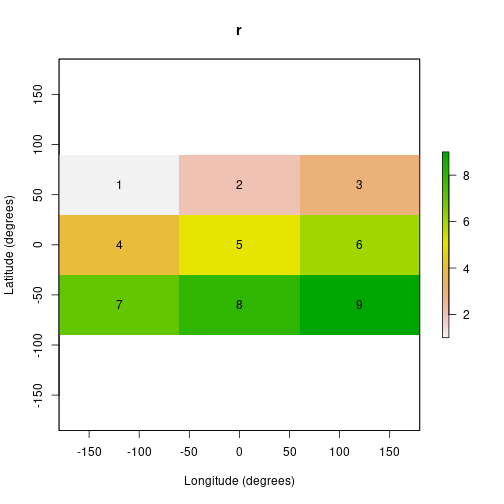# Transition* classes

As explained in Section 2 on the theory behind gdistance, transition matrices are the backbone of the package. The key classes in gdistance are TransitionLayer and TransitionStack. Most functions in the package have an object of one of these classes as input or output.

Transition* objects can be constructed from an object of class Raster*. A Transition* object takes the necessary geographic references (projection, resolution, extent) from the original Raster* object. It also contains a matrix which represents a transition from one cell to another in the grid. Each row and column in the matrix represents a cell in the original Raster* object. Row 1 and column 1 in the transition matrix correspond to cell 1 in the original raster, row 2 and column 2 to cell 2, and so on. For example, the raster we just created would produce a 9 x 9 transition matrix with rows and columns numbered from 1 to 9 (see Figure 3 below).

The matrix is stored in a sparse format, as discussed in Section 2. The package gdistance makes use of sparse matrix classes and methods from the package Matrix, which gives access to fast procedures implemented in the C language [@maechler2012]. The construction of a Transition* object from a Raster* object is straightforward. We can define an arbitrary function to calculate the conductance values from the values of each pair of cells to be connected.

In the following chunk of code, we use the RasterLayer that was created above. First, we set all its values to unit. The next line creates a TransitionLayer, setting the transition value between each pair of cells to the mean of the two cell values that are being connected. The directions argument is set to 8, which connects all cells to their 8 neighbours (Moore neighbourhood).

r[] <- 1
tr1 <- transition(r, transitionFunction=mean, directions=8)


If we inspect the object we created, we see that the resulting TransitionLayer object retains much information from the original RasterLayer object.

tr1
#> class      : TransitionLayer
#> dimensions : 3, 3, 9  (nrow, ncol, ncell)
#> resolution : 120, 60  (x, y)
#> extent     : -180, 180, -90, 90  (xmin, xmax, ymin, ymax)
#> crs        : +proj=longlat +datum=WGS84 +no_defs
#> values      : conductance
#> matrix class: dsCMatrix


To illustrate how to create an asymmetric matrix, we first create a non-commutative distance function, ncdf. We then use this function as an argument in the function transition. To make sure that the resulting transition matrix is indeed asymmetric, we set the symm argument in transition to FALSE.

r[] <- runif(9)
ncf <- function(x) max(x) - x + x
tr2 <- transition(r, ncf, 4, symm=FALSE)
tr2
#> class      : TransitionLayer
#> dimensions : 3, 3, 9  (nrow, ncol, ncell)
#> resolution : 120, 60  (x, y)
#> extent     : -180, 180, -90, 90  (xmin, xmax, ymin, ymax)
#> crs        : +proj=longlat +datum=WGS84 +no_defs
#> values      : conductance
#> matrix class: dgCMatrix


Note the difference between tr1 and tr2 in the slot ‘matrix class’. This slot holds information about the matrix class as defined in the package Matrix [@maechler2012]. The class dsCMatrix is for matrices that are symmetric. The class dgCMatrix holds an asymmetric matrix.

Different mathematical operations can be done with Transition* objects. This makes it possible to flexibly model different components of landscape friction.

tr3 <- tr1*tr2
tr3 <- tr1+tr2
tr3 <- tr1*3
tr3 <- sqrt(tr1)


Operations with more than one object require that the different objects have the same resolution and extent. Also, it is possible to extract and replace values in the matrix using indices, in a similar way to the use of indices with simple matrices.

tr3[cbind(1:9, 1:9)] <- tr2[cbind(1:9, 1:9)]
tr3[1:9, 1:9] <- tr2[1:9, 1:9]
tr3[1:5, 1:5]
#> 5 x 5 sparse Matrix of class "dgCMatrix"
#>
#> [1,] .         1.2890328 0.5303763 1.4784573 .
#> [2,] 0.2875775 .         0.4089769 .         1.0926294
#> [3,] 0.2875775 1.1676333 .         .         .
#> [4,] 0.2875775 .         .         .         0.9979172
#> [5,] .         0.7883051 .         0.8830174 .


The functions adjacent (from raster) and adjacencyFromTransition (from gdistance) can be used to create indices. Example 1 below illustrates this.

Some functions require that Transition* objects do not contain any isolated ‘clumps’, islands that are not connected to the rest of the raster cells. This can be avoided when creating Transition* objects, for instance by giving conductance values between all adjacent cells a small minimum value. It can be checked visually if there are any clumps. For the first method, the user can extract the transition matrix with function transitionMatrix. This gives a sparse matrix which can be vizualized with function image. This shows the rows and columns of the transition matrix and indicates which has a non-zero value, which represents a connection between cells (Figure 3).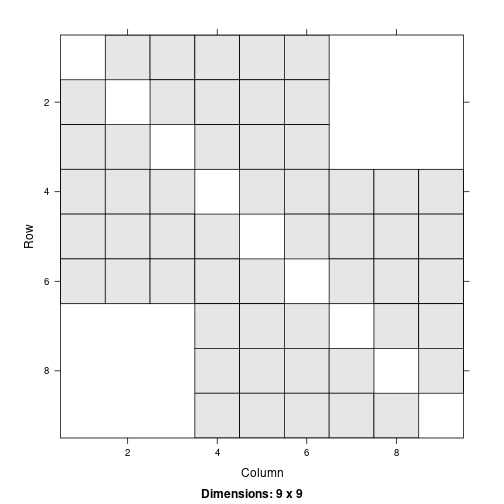Figure 3 shows which cells are connected to each other. A close observer of Figure 3 may wonder why cell 1 is connected to 5 different cells, as this cell is located in the upper left corner of the original grid. This is explained by the extent of this particular grid. Since it covers the whole world, the outer meridians (180 and -180 degrees) touch each other. The software takes this into account and as a result the cells in the extreme left column are connected to the extreme right column. If the grid does not reach across the globe in longitudinal direction, this does not occur.

Figure 3 shows which cells contain non-zero values, but gives no further information about levels of conductance. The levels can be visualized by transforming the transition matrix back into a raster. To summarize the information in the transition matrix, we can take means or sums across rows or columns. Users can do this with function raster. For the different options see method?raster("TransitionLayer"). The default, shown in Figure 4, takes the column-wise means of the non-zero values.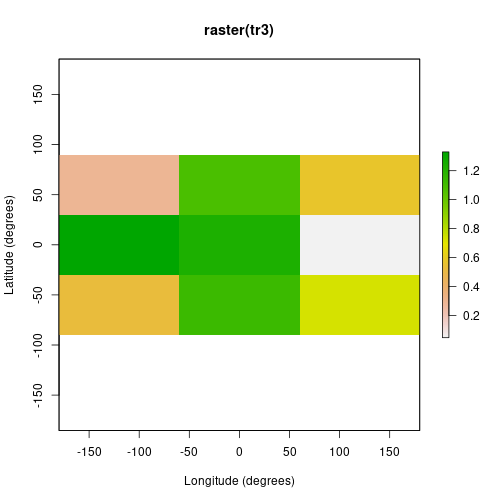# Correcting transition matrix values

The function transition calculates transition values based on the values of adjacent cells in the input raster. However, diagonal neighbours are more remote from each other than orthogonal neighbours. Also, on equirectangular (longitude-latitude) grids, West-East connections are longer at the equator and become shorter towards the poles, as the meridians approach each other.

Therefore, the values in the matrix need to be corrected for these two types of distance distortion. Both types of distortion can be corrected by dividing each conductance matrix value by the distance between cell centres. This is what function geoCorrection does when type is set to “c”.

tr1C <- geoCorrection(tr1, type="c")
#> as(<dsCMatrix>, "dgTMatrix") is deprecated since Matrix 1.5-0; do as(as(., "generalMatrix"), "TsparseMatrix") instead
tr2C <- geoCorrection(tr2, type="c")


However, as explained in Section 2 above, for commute distances (random walks) not only distance distortion plays a role, but also surface distortion. When type is set to “r” the function geoCorrection weights the probability of reaching an adjacent cell in a random walk by simply making it proportional to the surface covered by the cell. Computationally, the function corrects the surface distortion by multiplying the North-South transition values with the cosine of the average latitude of the two cell centres that are being connected.

r3 <- raster(ncol=18, nrow=9)
r3 <- setValues(r3, runif(18*9)+5)
tr3 <- transition(r3, mean, 4)
tr3C <- geoCorrection(tr3, type="c", multpl=FALSE, scl=TRUE)
tr3R <- geoCorrection(tr3, type="r", multpl=FALSE, scl=TRUE)


The argument scl is set to TRUE to scale the transition values to a reasonable range. If the transition values are too large, commute distance and randomized shortest path functions will not work well. No scaling should be done if the user wants to obtain absolute distance values as output.

In some cases, Transition* objects with equal resolution and extent need to be corrected many times. For example, determining the optimal landscape friction weights using a genetic algorithm involves repeating the same calculations with many transition matrices that only differ in their values, but not in their resolution or extent [@etten2010]. In this case, computational effort can be reduced by preparing an object that only needs to be multiplied with the Transition* object to produce a corrected version of it.

The following chunk of code is equivalent to the previous one.

CorrMatrix <- geoCorrection(tr3, type="r", multpl=TRUE, scl=TRUE)
tr3R <- tr3 * CorrMatrix


Object CorrMatrix is only calculated once. It can be multiplied with Transition* objects, as long as they have the same extent, resolution, and directions of cell connections. We need to take special care that the geo-correction multiplication matrix (CorrMatrix) contains all non-zero values that are present in the Transition* object with which it will be multiplied (tr3 in this case).

# Calculating distances

After obtaining the geographically corrected Transition* object, we can calculate distances between points. It is important to note that all distance functions require a Transition* object with conductance values, even though distances will be expressed in 1/conductance (friction or resistance) units (see Section 2 above).

To calculate distances, we need to have the coordinates of point locations. This is done by creating a two-column matrix of coordinates. Functions will also accept a SpatialPoints object or, if there is only one point, a vector of length two.

sP <- cbind(c(-100, -100, 100), c(50, -50, 50))


Calculating a distance matrix is straightforward now.

costDistance(tr3C, sP)
#>           1         2
#> 2 0.8842550
#> 3 0.9508077 1.5139996
commuteDistance(tr3R, sP)
#>           1         2
#> 2  998.0584
#> 3  980.4971 1085.3238
rSPDistance(tr3R, sP, sP, theta=1e-12, totalNet="total")
#>          [,1]     [,2]     [,3]
#> [1,]  0.00000 57.91140 56.75376
#> [2,] 56.62602  0.00000 62.12604
#> [3,] 55.76832 62.42598  0.00000


The costDistance function relies on the package igraph [@csardi2006] for the underlying calculation. It gives a symmetric or asymmetric distance matrix, depending on the TransitionLayer that is used as input.

Commute distance is the number of cells traversed during a random walk from a cell $$i$$ on the grid to a cell $$j$$ and back to $$i$$ [@chandra1996].

rSPDistance gives the cost incurred during the same walk ($\theta$ approaches zero, so this is the cost incurred during a random walk, see Section 2). To obtain the commute costs we sum the corresponding off-diagonal elements: $$d_{ij} + d_{ji}$$. This is the distance of a commute from $$i$$ to $$j$$ and back from $$j$$ to $$i$$.

In this case, the commute costs (resistance) are close to the commute distances (number of steps). This is because the TransitionLayer object has been scaled, so that transition costs are close to unit for each step and the total number of steps and the total distance are of the same order of magnitude.

# Dispersal paths

To determine dispersal paths of a (constrained) random walk, we use the function passage. This function can be used for both random walks and randomised shortest paths. The function calculates the average number of passages through cells or connections between cells before arriving in the destination cell. Either the total or net number of passages can be calculated. The net number of passages is the number of passages that are not reciprocated by a passage in the opposite direction. In other words, this is the probability of the “last forward” passage going through a cell-to-cell connection [@mcrae2008].

Figure 5 shows the net passages through each cell, assuming randomised shortest paths with the parameter $$\theta$$ set to 3.

origin <- SpatialPoints(cbind(0, 0))
rSPraster <- passage(tr3C, origin, sP[1,], theta=3)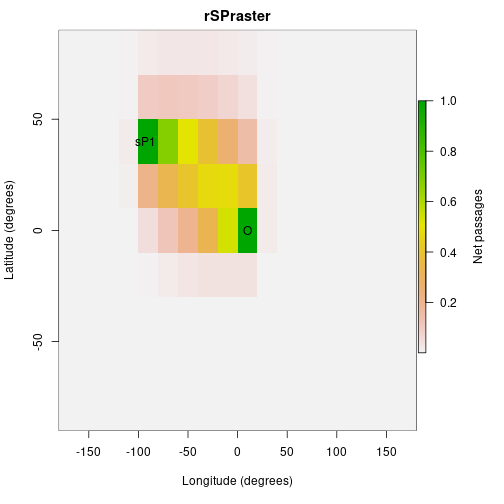# Path overlap and non-overlap

One of the specific uses for which package gdistance was created, is to look at dispersal trajectories of organisms that expand their range coming from a single source [@etten2010]. The degree of coincidence of two trajectories can be determined by calculating the minimum of the net passages of the two trajectories. With a formula presented in [@etten2010], we can approximate the non-overlapping part of the trajectory. This is done in the following code.

r1 <- passage(tr3C, origin, sP[1,], theta=1)
r2 <- passage(tr3C, origin, sP[2,], theta=1)
rJoint <- min(r1, r2)
rDiv <- max(max(r1, r2) * (1 - min(r1, r2)) - min(r1, r2), 0)


The first two lines create two different trajectories, both coming from the same point of origin (O) and going to different end destinations (referred to as sP1 and sP2 in the following). The third line obtains the minimum probability as a measure of the overlap between the two trajectories. The resulting raster rJoint is visualized in Figure 6. The fourth line calculates the non-overlapping part of the trajectories with a more complicated formula. The result, rDiv, is shown in Figure 7.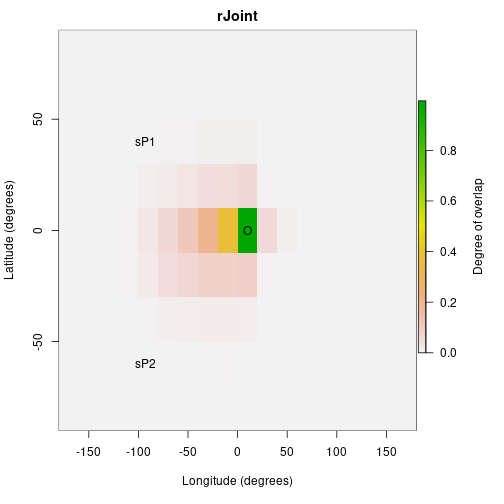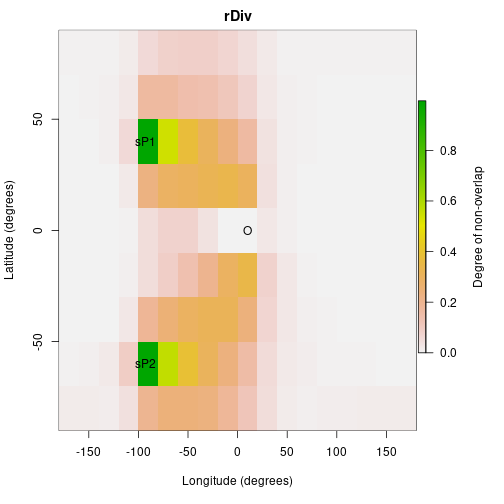With the function pathInc we can calculate measures of path overlap and non-overlap for a large number of points. These measures can be used to predict patterns of diversity if these are due to dispersal from a single common source. If the argument type contains two or more elements, the result is a list of distances matrices. The default for type is to calculate joint and divergent length of the dispersal paths.

pathInc(tr3C, origin, sP)
#> $function1layer1 #> 1 2 #> 2 2.363770 #> 3 2.318019 2.100372 #> #>$function2layer1
#>          1        2
#> 2 2.255957
#> 3 2.397766 2.802147


# Example 1: Hiking around Maunga Whau

The previous examples were theoretical, based on randomly generated values. More realistic examples serve to illustrate the various uses that can be given to this package.

Determining the fastest route between two points in complex terrain is useful for hikers. Tobler’s Hiking Function provides a rough estimate of the maximum hiking speed ($s$) given the slope of the terrain ($m$) [@tobler1993]. The maximum speed of off-path hiking (in m/s) is:

$$s = 6 e^{-3.5 |m + 0.05|}$$

Note that the function is not symmetric around 0 (see Figure 8). Hikers walk fastest on gently downward slopes ($m = -0.05$), where they can walk faster than on flat terrain ($m = 0$).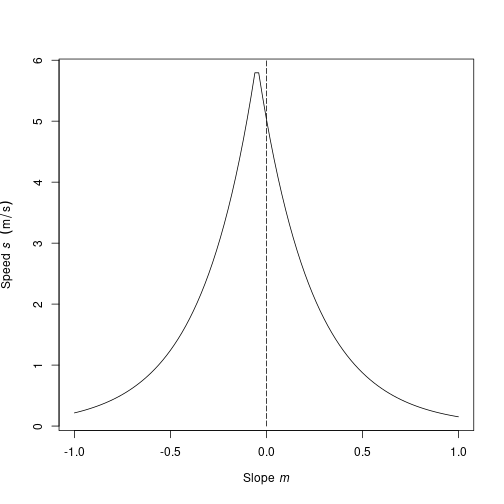We use the Hiking Function to determine the shortest path to hike around the volcano Maunga Whau (Auckland, New Zealand). First, we read in the altitude data for the volcano. This is the R base dataset (see ?volcano).

r <- raster(system.file("external/maungawhau.grd", package="gdistance"))


The Hiking Function requires the slope ($m$) as input, which can be calculated from the altitude ($z$) and distance between cell centres ($d$).

$$m_{ij} = (z_j - z_i) / d_{ij}$$

The units of altitude and distance should be identical. Here, we use meters for both. First, we calculate the altitudinal differences between cells. Then we use the geoCorrection function to divide by the distance between cells.

altDiff <- function(x){x - x}
hd <- transition(r, altDiff, 8, symm=FALSE)
#> Warning in .TfromR(x, transitionFunction, directions, symm): transition
#> function gives negative values

slope <- geoCorrection(hd)


The transition function throws a warning, because a matrix with negative values cannot be used directly in distance calculations. Here this warning can be safely ignored, however, as the negative values are only present in intermediate steps. Subsequently, we calculate the speed. We need to exercise special care, because the matrix values between non-adjacent cells is 0, but the slope between these cells is not 0! Therefore, we need to restrict the calculation to adjacent cells. We do this by creating an index for adjacent cells (adj) with the function adjacent.

Using this index, we extract and replace adjacent cells, without touching the other values.

adj <- adjacent(r, cells=1:ncell(r), pairs=TRUE, directions=8)
speed <- slope


Now we have calculated the speed of movement between adjacent cells. We are close to having the final conductance values. Attainable speed is a measure of the ease of crossing from one cell to another on the grid. However, we also need to take into account the distance between cell centres. Travelling with the same speed, a diagonal connection between cells takes longer to cross than a straight connection. Therefore, we use the function geoCorrection again.

Conductance <- geoCorrection(speed)


This gives our final ‘conductance’ values. What do these values mean? The function geoCorrection divides the values in the matrix between the distance between cell centres. So, with our last command we calculated conductance ($C$) as follows:

$$C = s / d$$

This looks a lot like a measure that we are more familiar with, travel time ($t$):

$$t = d / s$$

In fact, the conductance values we have calculated are the reciprocal of travel time ($1/t$).

$$1 / t = s / d = C$$

Maximizing the reciprocal of travel time is exactly equivalent to minimizing travel time. Distances calculated with this conductance matrix represent travel time according to the Hiking Function.

In the next step, we define two coordinates, A and B, and determine the paths between them. We test if the quickest path from A to B is the same as the quickest path from B back to A. The following code creates the shortest paths.

A <- c(2667670, 6479000)
B <- c(2667800, 6479400)
AtoB <- shortestPath(Conductance, A, B, output="SpatialLines")
BtoA <- shortestPath(Conductance, B, A, output="SpatialLines")


And this code reproduces Figure 9.

plot(r, xlab="x coordinate (m)", ylab="y coordinate (m)",
legend.lab="Altitude (masl)")
lines(AtoB, col="red", lwd=2)
lines(BtoA, col="blue")
text(A - 10, A - 10, "A")
text(B + 10, B + 10, "B")


A small part of the A-B (red) and B-A (blue) lines in Figure 9 do not overlap. This is a consequence of the asymmetry of the Hiking Function.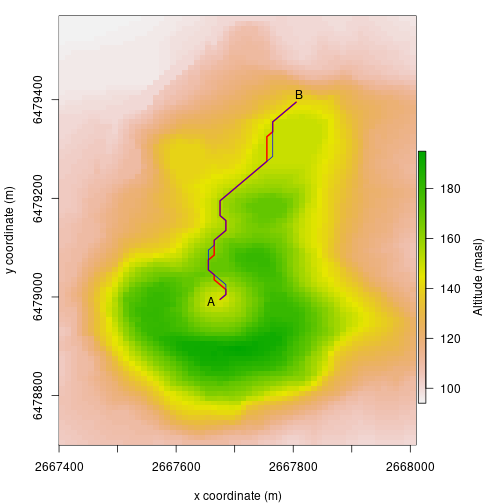# Example 2: Geographical genetics

A correlation between genetic differentiation and geographic distance of individuals and populations is expected due to a mechanism known as isolation by distance [@wright1943]. This correlation is expected when random, symmetric dispersal occurs in homogeneous geographic spaces. For random dispersal in heterogeneous landscapes, recent work has shown that genetic differentiation correlates with the resistance distance between their locations [@mcrae2006]. In this section, we look at human genetic diversity in Europe, using the data presented by [@bal2010].

First, we read in the data: a map of Europe, the coordinates of the populations (see Figure 10) and mutual genetic distances (see ?genDist for more information on these data).

Europe <- raster(system.file("external/Europe.grd",
package="gdistance"))
Europe[is.na(Europe)] <- 0
data(genDist)
data(popCoord)
pC <- as.matrix(popCoord[c("x","y")])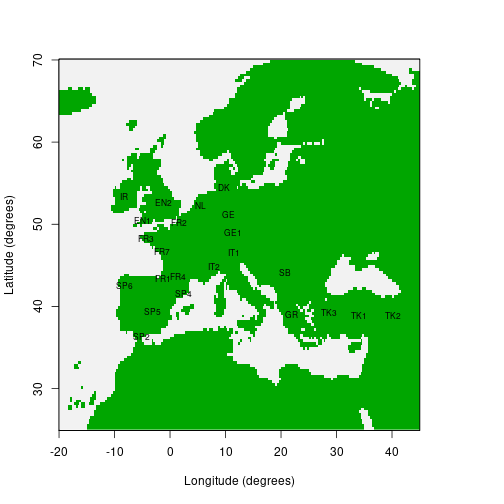Then we create three geographical distance matrices. The first corresponds to the great-circle distance between populations. The second is the least-cost distance between locations. Travel is restricted to the land mass. The third is the commute distance (using the same conductance matrix), which is related to effective resistance between points if we conceive of the grid as an electrical circuit [@chandra1996; @mcrae2006].

geoDist <- pointDistance(pC, longlat=TRUE)
geoDist <- as.dist(geoDist)
Europe <- aggregate(Europe,3)
tr <- transition(Europe, mean, directions=8)
trC <- geoCorrection(tr, "c", scl=TRUE)
trR <- geoCorrection(tr, "r", scl=TRUE)
cosDist <- costDistance(trC,pC)
resDist <- commuteDistance(trR, pC)
cor(genDist,geoDist)
#>  0.5964302
cor(genDist,cosDist)
#>  0.588961
cor(genDist,resDist)
#>  0.1917292


Among the distance measures evaluated until now, the great-circle distance between points turns out to be the best predictor of genetic distance. The other distance measures incorporate more information about the geographic space in which geneflow takes place, but do not improve the prediction. It follows that prehistoric people in Europe did not move like wolves (least-cost distance) or drunkards (commute or resistance distance), but rather like crows (great-circle distance).

An important assumption behind these distance measures, however, is that dispersal is symmetric. This is often not the case. For example, diffusion from a single origin (Africa) explains much of the current geographical patterns of human genetic diversity [@ram2005]. As a result, the mutual genetic distance between a pair of humans from different parts from the globe depends on the extent they share their prehistoric migration history. Within Europe, genetic diversity is often thought to be a result of the migration of early Neolithic farmers from Anatolia (now part of modern Turkey) to the west.

How well does a geographic wave of expansion from Anatolia explain the spatial pattern? The function pathInc calculates the overlap (and non-overlap) of dispersal paths from a common origin on the grid as a distance measure between points.

origin <- unlist(popCoord[22,c("x","y")])
pI <- pathInc(trC, origin=origin, from=pC,
functions=list(overlap))
cor(genDist,pI[])


At least at first sight, the overlap of dispersal routes explains the spatial pattern better than any of the previous measures. The negative sign of the last correlation coefficient was expected, as more overlap in routes is associated with lower genetic distance. Additional work would be needed to improve predictions and compare the different models more rigorously.

# Future work

Improvements of gdistance and methodological refinements are expected in various areas.

• All measures based on random walks depend critically on solving sparse linear systems. This is the most time-consuming part of the calculations. Faster libraries could improve the gdistance package if they become available in R` in the future.
• Research on distances in graph theory is a very dynamic field in the computational sciences. New measures and algorithms could be added to gdistance when they become available.
• More research on the consequences of connecting grids in different ways is necessary, as indicated in Section 2. This should bring more precision to random walk calculations in geospatial analysis. Comparing the results of grid-based calculations to continuous space simulations or analytical solutions would be the way forward [@birch2006].

# Acknowledgements

This research is supported by CGIAR Fund Donors. Work on the gdistance package started during the project “Collective Action for the Rehabilitation of Global Public Goods in the CGIAR Genetic Resources System: Phase 2” (2007-2010), under the guidance of Dr Robert Hijmans. Finalizing the package was undertaken as part of the CGIAR Research Program on Climate Change, Agriculture and Food Security (CCAFS), which is a strategic partnership of CGIAR and Future Earth. The views expressed in this document cannot be taken to reflect the official opinions of CGIAR or Future Earth.